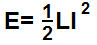﻿ Inductor Energy Calculator ﻿# Inductor Energy CalculatorpH nH µH H

A

0.0000000000J

The inductor energy calculator calculates the energy stored in an inductor, based on the size of the inductance of the inductor and the current going through it, according to the above formula.

A user enters the inductance, L, and the current, I, and the result will automatically be calculated and shown. The result which is displayed above is in unit Joules (J).

Calculating the amount of energy that an inductor stores can be useful in circuits containing inductors. Inductors may be used for various reasons in circuits, such as to form RL filter circuits. They are also used commonly after power supplies because inductors act as a surge protector in case there is a rush of current to a circuit. Since inductors resist the flow of current, they help to slow down this rush, which could destroy the circuits. In these types of circuits which use inductors, calculating the amount of energy that an inductor holds is important for a chief reason. Inductors have a characteristic called maximum DC current (IDC). Inductors can only pass through them a circuit amount of energy. If you know the amount of joules that an inductor energy field stores and you know the inductance of the inductor, you can use this to find out whether the amount of current which is passing through the inductor is at a safe level or not. The inductor energy level is also important because the amount of energy that an inductor stores determines how large in magnitude the magnetic field of the inductor will be. Inductors are devices which create a magnetic field when current passes through, when they are in a live circuit with live current. The greater the energy stored in the inductor, the greater the magnetic field, and conversely, the less energy stored is the lesser the magnetic field. Magnetic fields of inductors can be very important because the magnetic fields, if connecting with other circuit elements, can cause electromagnetic interference, which, in turn, can cause many undesirable interferences. Calculating the inductive energy can help to calculate the magnetic field. This can cause a circuit designer to be able to prevent this electromagnetic interference and build a EMI-free circuit. This is why knowing the energy that an inductor stores can be very important.

Related Resources

﻿